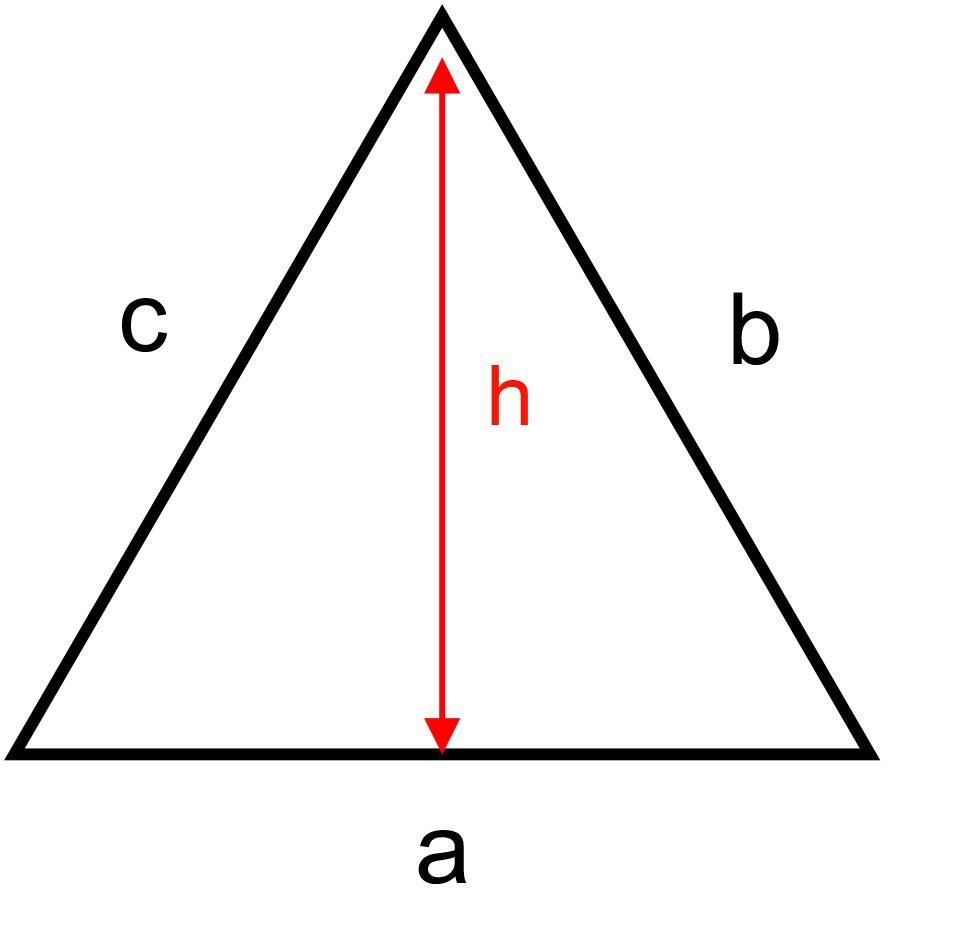﻿ Triangle surface: - on line calculation, formula - FORMIAX

# Triangle - surfaceS = (a * h)/2

S = surface area

a,b,c = sides

h = height

a = basement

WHAT IS IT ?

Triangle 3-sided polygon, flat shape with straight sides and 3 angles

CALCULATION:

Enter unit e.g.: inch

Enter triangle basement "a"

Enter triangle height "h"

Round to number of decimals places

YOU MIGHT BE INTERESTED: